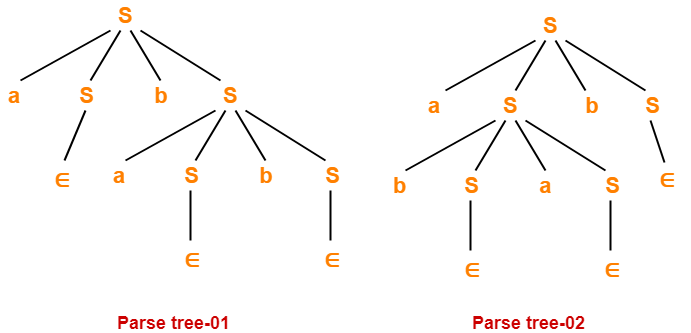# Algorithm To Decide Whether CFL Is Empty

## Context Free Language-

Before you go through this article, make sure that you have gone through the previous article on Context Free Language.

We have discussed-

• Context free language is generated using a context free grammar.
• Each context free language is accepted by a Pushdown automaton.

## Algorithm To Decide Whether CFL Is Empty Or Not-

 If we can not derive any string of terminals from the given grammar, then its language is called as an Empty Language. L(G) = ϕ

For a given CFG, there exists an algorithm to decide whether its language is empty L(G) = ϕ or not.

### Algorithm-

• Remove all the useless symbols from the grammar.
• A useless symbol is one that does not derive any string of terminals.
• If the start symbol is found to be useless, then language is empty otherwise not.

### Remember

The language generated from a CFG is non-empty iff the start symbol is generating.

## Example-

Consider the following grammar-

S → XY

X → AX

X → AA

A → a

Y → BY

Y → BB

B → b

Now, let us check whether language generated by this grammar is empty or not.

The given grammar can be written as-

S → aabb

X → aX

X → aa

A → a

Y → bY

Y → bb

B → b

Clearly,

• The start symbol generates at least one string (many more are possible).
• Therefore, start symbol is useful.
• Thus, language generated by the given grammar is non-empty.

 L(G) ≠ ϕ

## NOTE-

If L(G) = { ∈ } i.e.

• If the language generated by a grammar contains only a null string,
• then it is considered as non-empty.

Next Article- Algorithm To Decide Whether CFL Is Finite

Get more notes and other study material of Theory of Automata and Computation.

Watch video lectures by visiting our YouTube channel LearnVidFun.

SummaryArticle Name
Algorithm To Decide Whether CFL Is Empty
Description
Decision Properties of Context Free Languages and Decision Algorithms for Context Free Languages- Algorithm to decide whether a Context Free Language is empty or not.
Author
Publisher Name
Gate Vidyalay
Publisher Logo